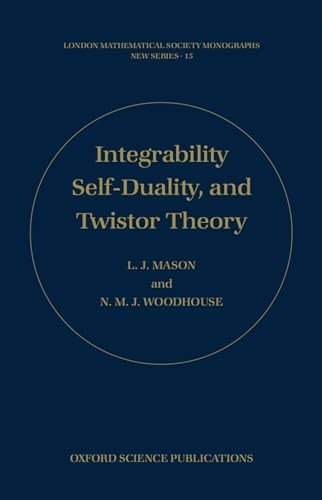# Integrability, Self-duality, and Twistor Theory by L. J. Mason (Mathematical Institute, Oxford)

In Stock
£105.99
This book explores in detail the connections between self-duality and integrability, and also the application of twistor techniques to integrable systems.
Only 1 left

## Integrability, Self-duality, and Twistor Theory Summary

### Integrability, Self-duality, and Twistor Theory by L. J. Mason (Mathematical Institute, Oxford)

It has been known for some time that many of the familiar integrable systems of equations are symmetry reductions of self-duality equations on a metric or on a Yang-Mills connection (for example, the Korteweg-de Vries and nonlinear Schroedinger equations are reductions of the self-dual Yang-Mills equation). This book explores in detail the connections between self-duality and integrability, and also the application of twistor techniques to integrable systems. It has two central themes: first, that the symmetries of self-duality equations provide a natural classification scheme for integrable systems; and second that twistor theory provides a uniform geometric framework for the study of Backlund tranformations, the inverse scattering method, and other such general constructions of integrability theory, and that it elucidates the connections between them.

### Why buy from World of BooksOur excellent value books literally don't cost the earthFree delivery in the UKEvery used book bought is one saved from landfill

## Integrability, Self-duality, and Twistor Theory Reviews

Anybody working in integrable systems or in twistor constructions will want a copy of this book or at least want it in their Library. * Proceedings of the Edinburgh Mathematical Society 1998, 41 *

PART I: SELF-DUALITY AND INTEGRABLE EQUATIONS; PART II: TWISTOR THEORY

GOR010203185
Integrability, Self-duality, and Twistor Theory by L. J. Mason (Mathematical Institute, Oxford)
L. J. Mason (Mathematical Institute, Oxford)
London Mathematical Society Monographs